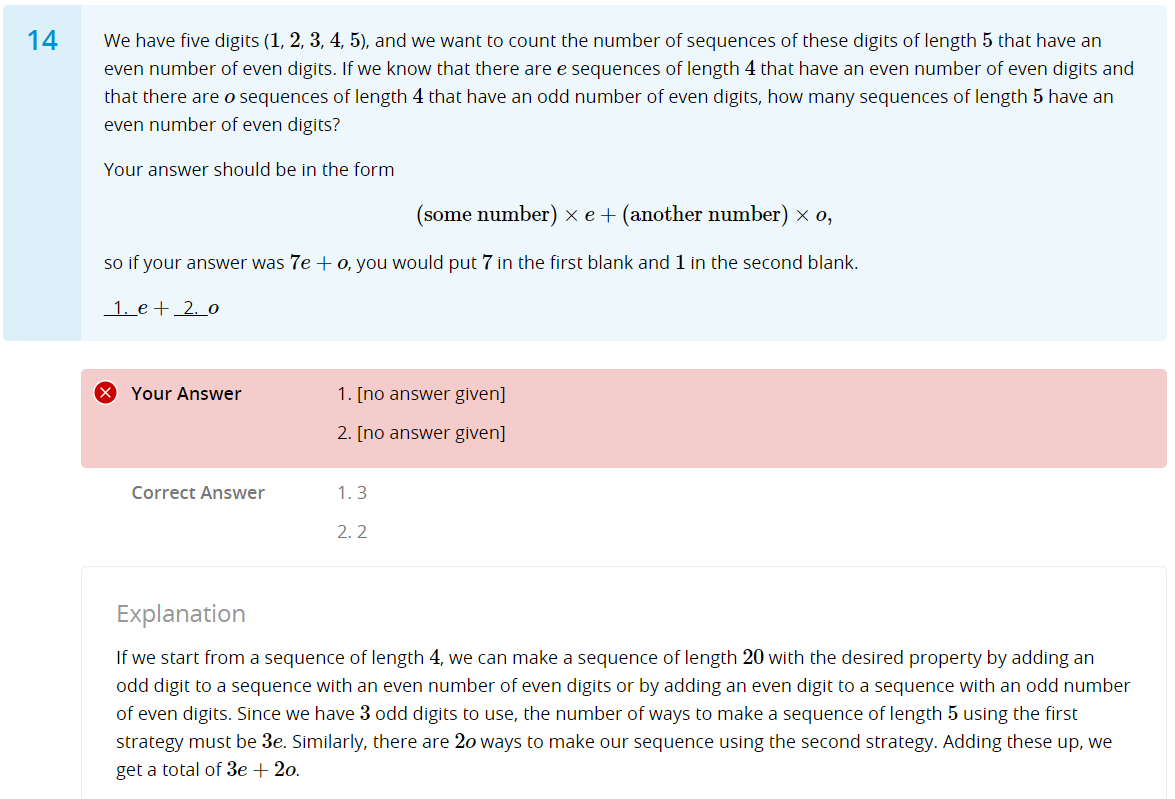# Module 3 Week 3 Challenge > Q14

• Hi everyone, I don't understand below question even after I read the explanation (Module 3 Week 3 Challenge > Q14), appreciate your help and advice. Thanks.• @modestwallaby Hi there!

So basically, we're making sequences from the digits 1-5 that are 5 digits long: for example, 12345, 45353, 15243. But, we want there to be an even number of even digits: this means that 12345 would work, since there are 2 even digits, but 45353 wouldn't (since there is only 1 even digit).

Now, the question is assuming that we know the number of sequences that are 4 digits long that have an odd number of even digits: whatever this number is, we assign it to a variable e. Similarly, for some number o, there are o sequences that are 4 digits long and have an even number of even digits.

We want to figure out the number of 5-length sequences with an even number of even digits, in terms of e and o. This is a recursion problem, and the key question is: how can you construct a valid 5-length sequence from some 4-length sequence?

Well, we can do that by adding on a digit to a 4-length sequence. There are 2 cases here: either the 4-length sequence has an even number of even digits, or it has an odd number of even digits.

Case 1: 4-length sequence with even number of even digits
By the problem's conditions, there are e sequences that satisfy this case. And now we want to build a 5-length sequence with an even number of even digits. That means the 5th digit that we add on must be odd! (If the 5th digit was even, we would have even + 1 = odd number of even digits). In other words, the 5th digit needs to be 1, 3, or 5.
This means there are 3e total possibilities for this case (e starting sequences, 3 ways to add on a digit to each sequence).

Case 2: 4-length sequence with odd number of even digits
Again, based on what the problem tells us, there are o sequences that satisfy this case. And in this case, we need the 5th digit to be even, so that the total number of even digits is odd + 1 = even. Thus, the 5th digit has to be 2 or 4.
There are 2o total possibilities here (o starting sequences, 2 ways to add on a digit to each sequence).

Thus, the total number of possible sequences is just the sum of what we got for each case = 3e + 2o.

I hope that helped, and let me know if there's something I could clarify!• @audrey Thanks a lot!